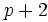# Difference between revisions of "Twin prime conjecture"

View other infinitude conjectures | View infinitude facts

## Statement

There are infinitely many twin primes. In other words, there are infinitely many odd primessuch thatis also a prime.

In other words, the limit inferior of all prime gaps is.

## Relation with other conjectures and known facts

### Limit inferior of prime gaps

The twin primes conjecture can be viewed as saying that the lim inf of prime gaps is. For more results on the current state of the art in knowing of the limit inferior of prime gaps (both unconditional and conditional to various hypotheses) see the prime gap page.

### Average prime gap

The prime number theorem states that the average prime gap is the natural logarithm of the prime.

### Supremum of prime gaps

• Bertrand's postulate (which is in fact a theorem) states that there always exists a prime between any number and its double.

### Generalizations

• Schinzel's hypothesis H is a much stronger and more general conjecture that provides a framework within which the twin primes conjecture fits.